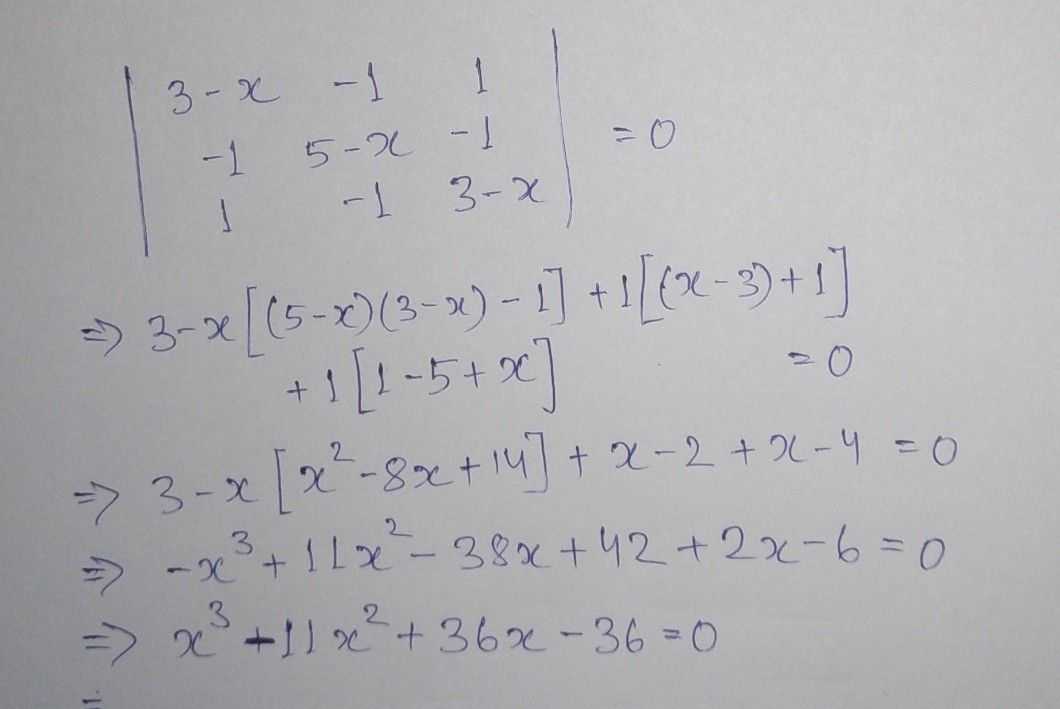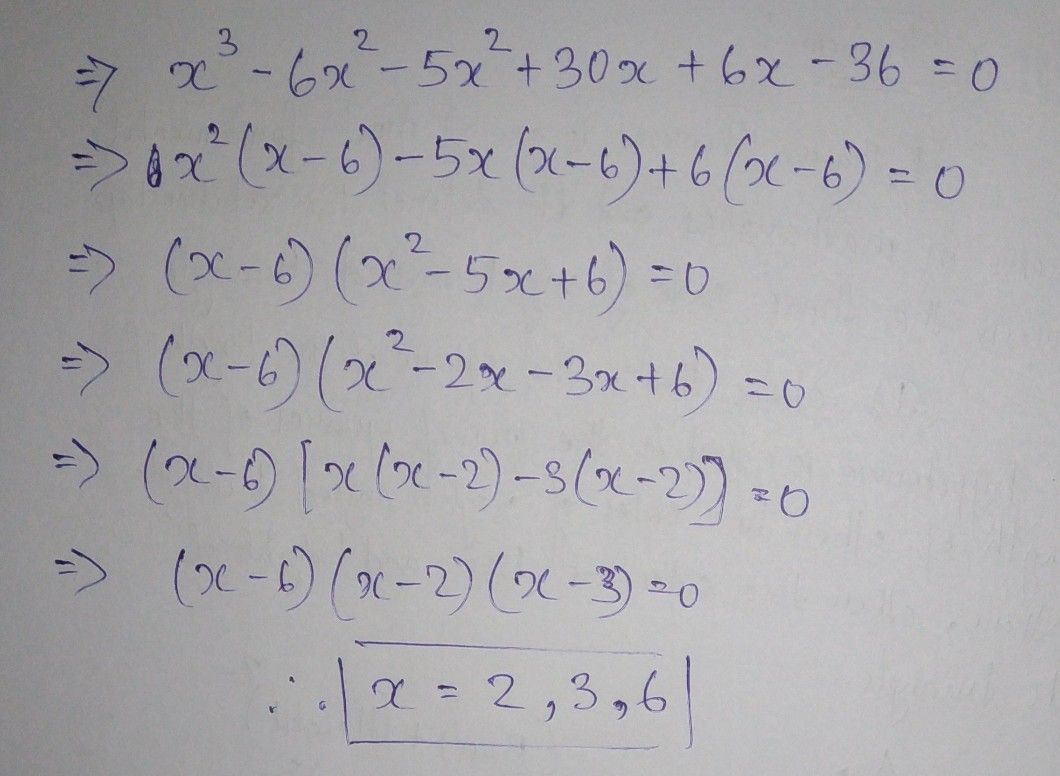Symbol
Problem$3-x$ $-1$ $1$ $-1$ $5-x$ $-1$ $1$ $=0.$ $1$ $-1$ $3-x$ $|$
10th-13th grade
Other
SolutionQanda teacher - BhanuStudent
it has to solved using properties of determinantsQanda teacher - Bhanu
that was not mentioned anywhere.
So, please mention it ask a different problem separately.